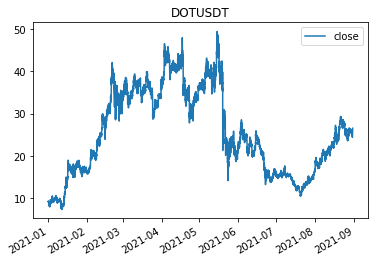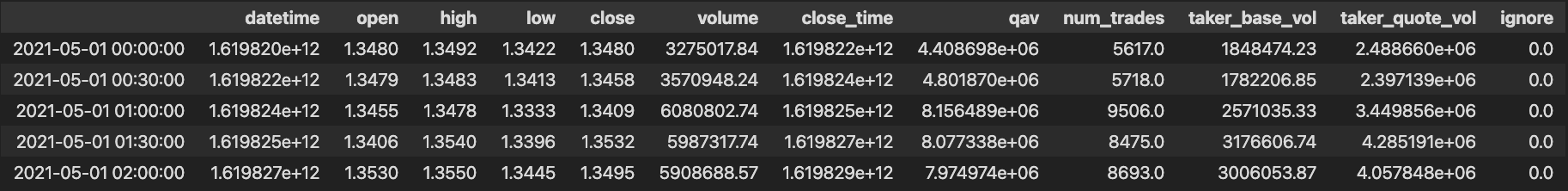In the age of data-driven investing, it is necessary to have access to quality data, luckily we can do that by simply using python and Binance.

There are two options to do this:

1. use a python package called python-binance

2. Use the python requests library to get data from binance

If you don’t have an account you can register here to get a 10% fee discount https://accounts.binance.com/en/register?ref=WUN0PLU5

1. Let’s go over the first option: Python Binance

First, we will install Python Binance with pip: https://github.com/sammchardy/python-binance

Let’s import all the necessary libraries and initialize the client

``````import pandas as pd
from binance.client import Client
import datetime as dt

# client configuration
api_key = 'API HERE'
api_secret = 'SECRET API HERE'
client = Client(api_key, api_secret)
``````

Now we will select the symbol name(uppercase) and the time interval. Next we will pass the data that we got into a pandas DataFrame and call it data. In this step the index and columns name will be formatted. Finally, we will save the df into a CSV with “data.to_csv(‘filename’)” and plot the data to see what we got!

``````symbol = "DOTUSDT"
interval=’15m’

Client.KLINE_INTERVAL_15MINUTE

klines = client.get_historical_klines(symbol, interval, "1 Jan,2021")

data = pd.DataFrame(klines)
# create colums name
data.columns = ['open_time','open', 'high', 'low', 'close', 'volume','close_time', 'qav','num_trades','taker_base_vol',taker_quote_vol', 'ignore']

# change the timestamp
data.index = [dt.datetime.fromtimestamp(x/1000.0) for x in data.close_time]

#convert data to float and plot

df=df.astype(float)
df["close"].plot(title = 'DOTUSDT', legend = 'close')
``````PLOT FOR DOTUSDT

1. PYTHON REQUESTS

Let’s import all the libraries needed

``````import requests
import json
import pandas as pd
import datetime as dt
``````

We will initialize the symbol that we want to retrieve data for, the interval, the start and end dates. These parameters can be found on the official Binance API docs at: https://binancedocs.github.io/apidocs/spot/en/#change-log

``````url = 'https://api.binance.com/api/v3/klines'
interval = '1h'
start = str(int(dt.datetime(2021,5,1).timestamp()*1000))
end = str(int(dt.datetime(2021,8,1).timestamp()*1000))

par = {'symbol': symbol, 'interval': interval, 'startTime': start, 'endTime': end}

#format columns name

data.columns = ['datetime', 'open', 'high', 'low', 'close', 'volume','close_time', 'qav', 'num_trades','taker_base_vol', 'taker_quote_vol', 'ignore']

data.index = [dt.datetime.fromtimestamp(x/1000.0) for x in data.datetime]

data=data.astype(float)
``````

Let’s show the first rows of the datafram with data.head()